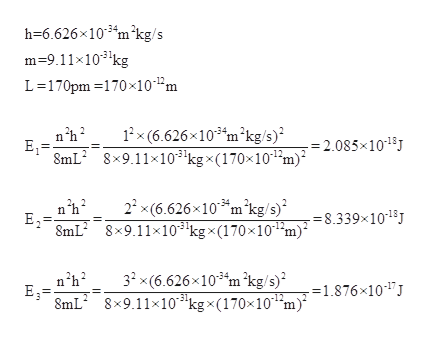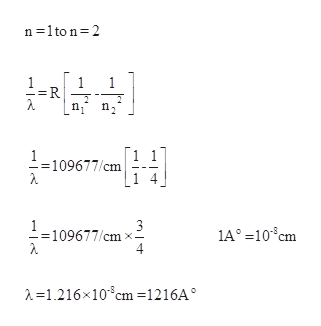An electron confined to a one-dimensional box has energy levels given by the equationEn=n2h2/8mL2where n is a quantum number with possible values of 1,2,3,…;m is the mass of the particle; and L is the length of the box. a. Calculate the energies of the n=1,n=2, and n=3 levels for an electron in a box with a length of 170 pm .b. Calculate the wavelength of light required to make a transition from n=1→n=2 and from n=2→n=3.c.In what region of the electromagnetic spectrum do these wavelengths lie?radiomicrowaveinfraredvisible lightultravioletX-raygamma ray

Question

An electron confined to a one-dimensional box has energy levels given by the equation

En=n2h2/8mL2

where n is a quantum number with possible values of 1,2,3,…;m is the mass of the particle; and L is the length of the box.

a. Calculate the energies of the n=1,n=2, and n=3 levels for an electron in a box with a length of 170 pm .

b. Calculate the wavelength of light required to make a transition from n=1→n=2 and from n=2→n=3.

c.
In what region of the electromagnetic spectrum do these wavelengths lie?
 radio microwave infrared visible light ultraviolet X-ray gamma ray

Step 1

a. An electron confined to a one-dimensional box has energy levels given by the equation

Step 2

The energy of each level is calculated as,help_outlineImage Transcriptioncloseh-6.626x103 m2kg/s m9.11x103kg L-170pm 170x10^2m 1x (6.626x103 m2kg/s) 8mL2 8x9.11x103'kgx(170x101m) 2.085x101 E1 22 x(6.626x103m2kg/s) 8mL2 8x9.11x10 kgx(170x10 8.339x1013 E2 12 32 x(6.626x103 m 'kg/s) 8x9.11x103kgx(170x10 m1.876x10 E 8mL -12 fullscreen
Step 3

b. The wavelength required for tran...help_outlineImage Transcriptionclosen 1to n 2 1 R 2 1 1 109677/cm 3 109677/cm 4 1A°-10cm -1.216x10m =1216A° fullscreen

Want to see the full answer?

See Solution

Want to see this answer and more?

Our solutions are written by experts, many with advanced degrees, and available 24/7

See Solution
Tagged in

General Chemistry